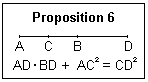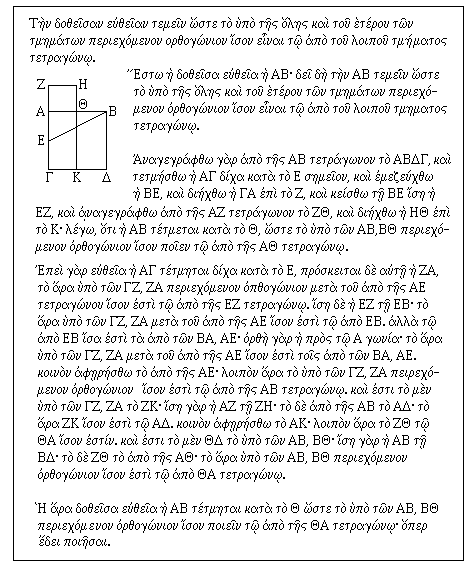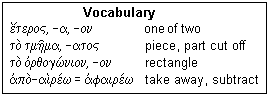## Lesson κα': Book II, Proposition 11

This proposition shows how to construct the Golden Section

This proposition is an example of the geometrical algebra that makes up most of the Elements. Book II is the first devoted to this subject, where solutions to some problems required elsewhere in the Elements are found. There are 14 propositions in Book II, all problems. The proposition we treat here is used directly to inscribe an equilateral pentagon in a circle (Book IV, Proposition 11), in the construction of an angle of 72° (Book IV, Proposition 10). It is certainly not an obvious result, as are all the others that we have so far met. A quick and easy graphical construction can be derived from the problem. Graphical solutions were very popular in the ancient world, especially in engineering and construction, and are very effective. Most current observers are unaware of their power, though they were commonly used in engineering until quite recently.The problem is to divide a line segment so that the product of the whole line and one of the segments is equal to the square of the other segment. That is, if AB is the given line, and C is a point on it, we want to locate C so that AB x AC = CB x CB. This can be rearranged to read CB/AC = AC/AB, or "the smaller is to the larger as the larger is to the whole." This is called the Golden Section, a proportion thought ideal by Renaissance theorists. A rectangle with these proportions is shown at the right. Algebraically, if the length of the line is taken as unity, and x is one segment, then x = (1 - x)2, or x2 - 3x + 1 = 0. The solution less than 1 is x = (3 - √5)/2 = 0.382. The other segment is then 1 - 0.382 = 0.618, and the ratio of the two is 1.618.The proof of this proposition rests on an earlier proposition, Proposition 6, which states: "If a straight line be bisected, and produced to any point, the rectangle contained by the whole line thus produced, and the part of it produced, together with the square on half the line bisected, is equal to the square on the straight line which is made up of the half and the part produced." This property is shown in the box on the right, in algebraic terms. This proposition is also not exactly obvious, but you can easily show that it is true using algebra.The proposition is shown at the right, and we have a small vocabulary box below. Again, there are few new words, and most of the others we have already met in Proposition 47. The statement is: "The given straight line to be cut so that the rectangle enclosed by the whole and one of the two segments to be equal to the square on the remaining segment." We proceed with the application to the figure: "Let AB be the given line. It is necessary to cut AB so the rectangle enclosed by the whole and one of the two segments be equal to the square on the remaining segment." We cannot go further with this until we have made some constructions, which we do in the next paragraph: "Describe the square ABΔΓ on AB, and let AΓ be bisected by the point E, and join BE, and extend ΓA to Z, and lay off EZ equal to BE, and describe the square ZΘ on AZ, and extend HΘ to K; I say, that AB is cut by Θ so that the rectangle enclosed by AB, BΘ is made equal to the square on AΘ" There is a long string of ands connecting the successive steps, but we finally were able to state the theorem in terms of the figure.

Now to the proof: "For since the straight line AΓ is bisected by E, added to which is ZA, therefore the rectangle enclosed by ΓZ, ZA with the square on AE, is equal to the square on EZ." We have just used Proposition 6. Proceeding, "And EZ is equal to EB; Therefore the [rectangle] on ΓZ, ZA with the [square] on AE is equal to the [square] on EB. But the [square] on EB is equal to the [squares] on BA, AE; for the angle at A is a right angle; Therefore, the [rectangle] on ΓZ, ZA with the [square] on AE is equal to the [squares] on BA, AE. Take away the common [square] on AE. Therefore, the remaining rectangle enclosed by ΓZ, ZA is equal to the square on AB. And the [rectangle] on ΓZ, ZA is the [rectangle] ZK; for AZ is equal to ZH; and the [square] on AΔ is up on AB. Therefore, ZK is equal to AΔ. Take away the common [rectangle] AK; Therefore, the remainder ZΘ is equal to ΘA. And ΘA is that on AB, BΘ; for AB is equal to BΔ; and ZΘ to the [square] on AΘ; Therefore, the rectangle enclosed by AB, BΘ is equal to the square on ΘA." This is all pure algebra, done with the figure and in words. We have had to supply "square" and "rectangle" where Euclid resorted to a shorthand to avoid tedious repetition.

Now for the summing-up: "Therefore, the given straight line AB is cut by Θ so that the rectangle enclosed by AB, BΘ to be made equal to the square on ΘA; which was to be done." Unusually, this does not closely reproduce the words used to state the proposition at the beginning. However, it does seem a little more straightforward.The vocabulary is shown at the left. e/(teros refers to one of two, either this one or that one. The line is divided into two segments, so either one can be called e/(teron. The word for segment, or thing cut off, is a standard neuter. "Orthogon" is the word for rectangle, referring to its right angles. Finally, the word for "take away" literally means away-take. Note that the ο is elided, and the π before the rough breathing changes to φ. Any augment will take place after the prefix, as in the aorist participle in the text. This is the source of the iota-subscript in the middle of the word. The accented ε before the ω shows that the verb will usually contract. The little word w/(ste means "so that", of course.